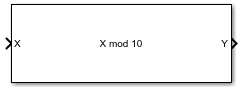Modulo by Constant

Perform modulo operation with a constant denominator

• Library:
• Fixed-Point Designer

•Description

The Modulo by Constant block performs the modulo operation (remainder after division) with a constant denominator.

The Modulo by Constant block uses an algorithm that is functionally similar to a Barrett Reduction. The division operation is computed via a multiplication by inverse, which generally results in better performance on embedded systems.

Ports

Input

expand all

Dividend, specified as a real scalar.

If X is a fixed-point data type, it must use binary-point scaling.

Data Types: single | double | int8 | int16 | int32 | int64 | uint8 | uint16 | uint32 | fixed point

Output

expand all

Result of modulus operation, returned as a scalar.

Data Types: single | double | int8 | int16 | int32 | int64 | uint8 | uint16 | uint32 | fixed point

Parameters

expand all

Divisor to use for the modulus operation, specified as a positive, real-valued, finite scalar.

Programmatic Use

 Block Parameter: Denominator Type: character vector Values: MATLAB® expression that evaluates to a positive, real-valued, finite fixed point or numeric value Default: '10'

Data Types: single | double | int8 | int16 | int32 | int64 | uint8 | uint16 | uint32 | uint64 | Boolean | fixed point

Extended Capabilities

Introduced in R2021a

Fixed-Point Designer DocumentationGet trial now